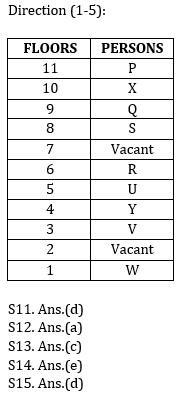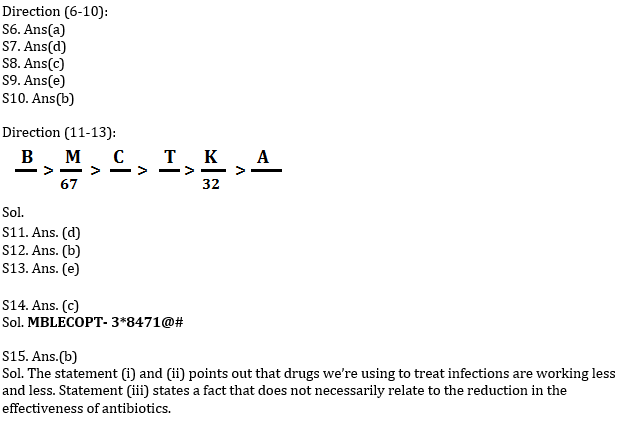# Reasoning Ability Quiz for IBPS 2020 Mains Exams- 11th December

Directions (1-5): Study the given information and answer the following questions.
Nine persons U, V, W, X, Y, P, Q, R and S are living on an eleven floor building such as ground floor is numbered as 1, just above floor is numbered as 2 and so on till the topmost floor is numbered as 11 where two floors remained vacant. All the information is not necessarily in the same order.
Three persons live between X and U. No one lives between V and W. U lives immediately above Y’s floor. No one lives above the P’s floor. W lives on the ground floor. R lives on an even numbered floor but above the 4th floor. The number of persons live between the two vacant floors is same as the number of persons live between S and the one who lives on ground floor. Q lives above the vacant floors. Vacant floors are not adjacent to each other. Not more than two floors gap between R and V. Y lives below the R’s floor.

Q1. Which among the following floor is vacant floor?
(a) 7th floor
(b) 11th floor
(c) 2nd floor
(d) Both (a) and (c)
(e) Both (b) and (C)

Q2. How many persons are living between P and V?
(a) Six
(b) Seven
(c) Five
(d) Four
(e) None of these

Q3. X lives on which among the following floor?
(a) 4th floor
(b) 6th floor
(c) 10th floor
(d) 2nd floor
(e) None of these

Q4. Four of the following are alike in a certain way so form a group, which of the following does not belong to that group?
(a) P
(b) Q
(c) U
(d) W
(e) R

Q5. How many floors are there between Q and W’s floor?
(a) Five
(b) Three
(c) Four
(d) Seven
(e) None of these

Directions (6-10): In the following questions, the symbols #, \$, %, &, and @ are used with the following meaning as illustrated below:
‘Q # P’ means ‘Q is not greater than P’.
‘Q \$ P’ means ‘Q is neither smaller than nor equal to P.
‘Q % P’ means ‘Q is neither smaller nor greater than P’
‘Q & P’ means ‘Q is neither greater than nor equal to P’.
‘Q @ P’ means ‘Q is not smaller than P’.

Now in each of the following questions, assuming the given Statements to be true, find which of the two conclusions I and II given below them is/are definitely true and give your answers accordingly. Mark answer

Q6. Statements :
Z # Y,    Y % X,   X &  W
Conclusion:
I.
X @ Z
II.
Z # W
(a) If only conclusion I is true.
(b) If only conclusion II is true.
(c) If either conclusion I or II is true.
(d) If neither conclusion I nor II is true.
(e) If both conclusions I and II are true.

Q7. Statements :
K \$ J,      J @ H,    H % I
Conclusion:
I. K @ I
II. I \$ J
(a) If only conclusion I is true.
(b) If only conclusion II is true.
(c) If either conclusion I or II is true.
(d) If neither conclusion I nor II is true.
(e) If both conclusions I and II are true.

Q8. Statements :
D &  C, C # B,    B \$ A
Conclusion:
I. D & A
II. D @A
(a) If only conclusion I is true.
(b) If only conclusion II is true.
(c) If either conclusion I or II is true.
(d) If neither conclusion I nor II is true.
(e) If both conclusions I and II are true.

Q9. Statements :
T @ S,   S \$ Q,     Q % P
Conclusion:
I. P & T
II. Q & T
(a) If only conclusion I is true.
(b) If only conclusion II is true.
(c) If either conclusion I or II is true.
(d) If neither conclusion I nor II is true.
(e) If both conclusions I and II are true.

Q10. Statements :
M @ N, N \$ K,    K # G
Conclusion:
I. G # M
II. K & M
(a) If only conclusion I is true.
(b) If only conclusion II is true.
(c) If either conclusion I or II is true.
(d) If neither conclusion I nor II is true.
(e) If both conclusions I and II are true.

Directions (11-13): Study the following information carefully and answer the questions given below:
Six persons are arranged according to their weights in descending order from left to right. At most 2 persons are heavier than C. The weight of 2nd lightest person is 32kg. M is heavier than T but lighter than B. M is heavier than C. One person arranged between T and the one whose weight of 67kg. A is not heavier than T. T is heavier than K, who is not the lightest among all.

Q11. How many persons are heavier than T?
(a) One
(b) None of these
(c) Two
(d) Three
(e) More than three

Q12. What may be the possible weight of C?
(a) 70kg
(b) 49kg
(c) 30kg
(d) 31kg
(e) 68kg

Q13. How many persons are heavier than K?
(a) One
(b) None
(c) Three
(d) Two
(e) Four

Q14. If COMPUTER is coded as ‘713@2#46’ and MOBILE is coded as ‘31*584’, then how will ‘MBLECOPTis coded as?
(a) 3*8417@#
(b) 3*8147@#
(c) 3*8471@#
(d) 3*8471#@
(e) 3*8741#@

Q15. Statement- According to the Director-General of the World Health Organization “The world is heading towards a post-antibiotic era in which common infections will once again kill. If current trends continue, sophisticated interventions, like organ transplantation, joint replacements, cancer chemotherapy, and care of pre-term infants, will become more difficult or even too dangerous to undertake. This may even bring the end of modern medicine as we know it.”
Which of the following statement substantiates the views expressed by the Director General of WHO?
(i) The efficacy of the world’s antibiotics is quickly decaying.
(ii) Bacteria are becoming resistant to modern day medicines.
(iii) A 2015 study published in Nature found that global antibiotic consumption went up 30% between 2000 and 2010.
(a) Only (i)
(b) Both (i) and (ii)
(c) Only (i) and (iii)
(d) All (i),(ii) and (iii)
(e) None of these

Practice More Questions of Reasoning for Competitive Exams:

###### Prelims Quiz Study Plan for SBI & IBPS Exams 2020

SolutionsPractice with Crash Course and Online Test Series for IBPS Mains 2020: